# A guide to using colors in R

There are three types of R colors:

• hexadecimal colors (#rrggbb) or (#rrggbbaa) aa being alpha (how opaque the color is)
• named colors e.g. (red, blue, purple, etc.)
• integers referring to positions in the current color palette

Colors are graphic parameters described in the `par()` documentation of the graphics library.

NA Specify a hexadecimal color as a parameter by placing the name within quotes, such as

``barplot(1, axes=FALSE, col="#4682B4")``The alpha parameter can be used to de ne tranparency. Simply add two more digits, in the form `#rrggbbaa`.

``barplot(1, axes=FALSE, col="#4682B433")  ``# Named colors

R can interpret hundreds of named colors, such as `"plum"`, `"seagreen2"`, and `"peachpuff3"` as hexadecimal colors. To see a list of the named colors (just the names, not the colors themselves) use the command `colors()`.

Use this code to view the rgb values for all named colors:

``````crgb <- col2rgb(cc <- colors())
colnames(crgb) <- cc
``````              red green blue
white         255   255  255
aliceblue     240   248  255
antiquewhite  250   235  215
antiquewhite1 255   239  219
antiquewhite2 238   223  204
antiquewhite3 205   192  176``````

Specify a named color as a parameter by placing the name within quotes, such as:

``barplot(1, axes=FALSE, col="steelblue")``# The color palette

The `palette()` function within the grDevices library allows a table of colors to be referenced by a numeric index. The default color palette is:

1 = “black”
2 = “red”
3 = “green3”
4 = “blue”
5 = “cyan”
6 = “magenta”
7 = “yellow”
8 = “gray”

To set these colors as parameters, simply use the index:

``barplot(1, axes=FALSE, col=4)````barplot(c(1, 1, 1), axes=FALSE, col=c(4,5,6))``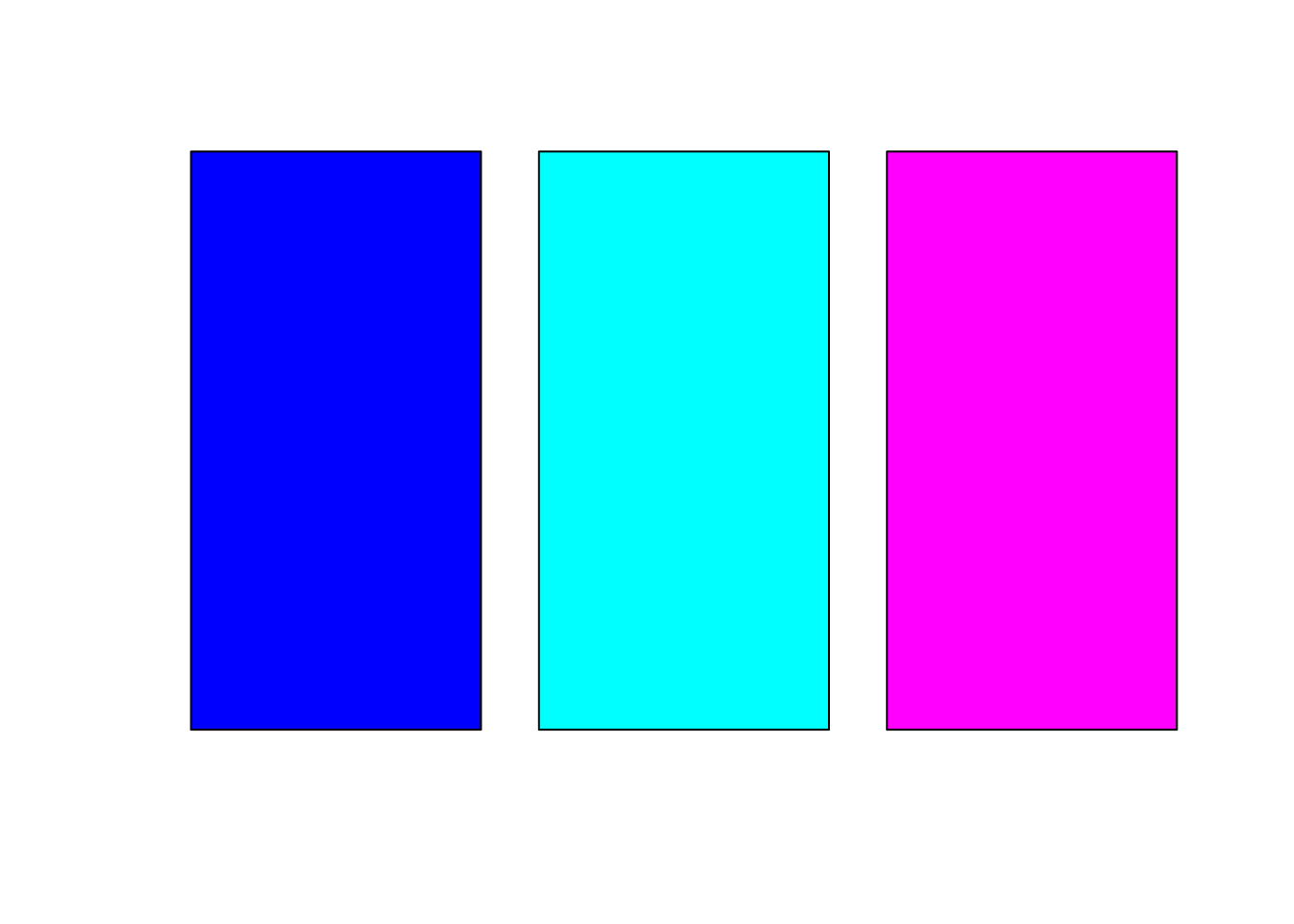The color palette can be changed by providing a vector of colors:

``````palette(c("red", "#4682B4", "#00008B", "darkgreen"))
barplot(c(1,1,1,1), axes=FALSE, col=c(1,2,3,4))``````View the current palette with `palette()`.
Return to the default palette with `palette("default")`.

# Converting rgb to hex color

The `rgb()` function converts red, green, and blue intensities to a hexidecimal representation. The function has the form `rgb(red, green, blue, alpha, names = NULL, maxColorValue = 1)`

alpha is an optional argument for transparency, and has the same intensity scale as the red, green, and blue values. names is an optional argument that will print a name with the hexadecimal value. NA

``````new_orange = rgb(255, 127, 0, maxColorValue=255)
barplot(1, axes=FALSE, col=new_orange)``````# Converting an R color to rgb

The `col2rgb()` function converts R colors (a hexadecomal color, named color, or integer representing a palette position) to the rgb representations. The function takes either a single color or a vector of colors, and returns a matrix of three rows (red, green, blue), with one column for each color. The function has the form `col2rgb(color, alpha=FALSE)`
alpha is an optional argument to indicate whether alpha transparency values should be returned. Converting a single color to rgb:

``col2rgb("steelblue")``
``````      [,1]
red     70
green  130
blue   180``````

Converting a vector of colors to rgb:

``col2rgb(c("#4682B433", "#104E8b", "mistyrose"))``
``````      [,1] [,2] [,3]
red     70   16  255
green  130   78  228
blue   180  139  225``````

Converting a vector of colors to rgb, with labels in matrix columns:

``col2rgb(c(orange="#4682B433", blue="#104E8b", pink="mistyrose"))``
``````      orange blue pink
red       70   16  255
green    130   78  228
blue     180  139  225``````

# Creating a vector of grays

The `gray.colors()` function creates a vector of evenly-spaced gray colors. The function has the form
`gray.colors(num_colors, start=value, end=value, gamma=value)`
end and start are used to specify the endpoints of the range of grays, with 0 = black and 1 = white. (By default, start=0.3 and end=0.9.)
gamma is an optional argument for gamma correction.

``barplot(rep(1,100), col = gray.colors(100, start =1, end = 0))``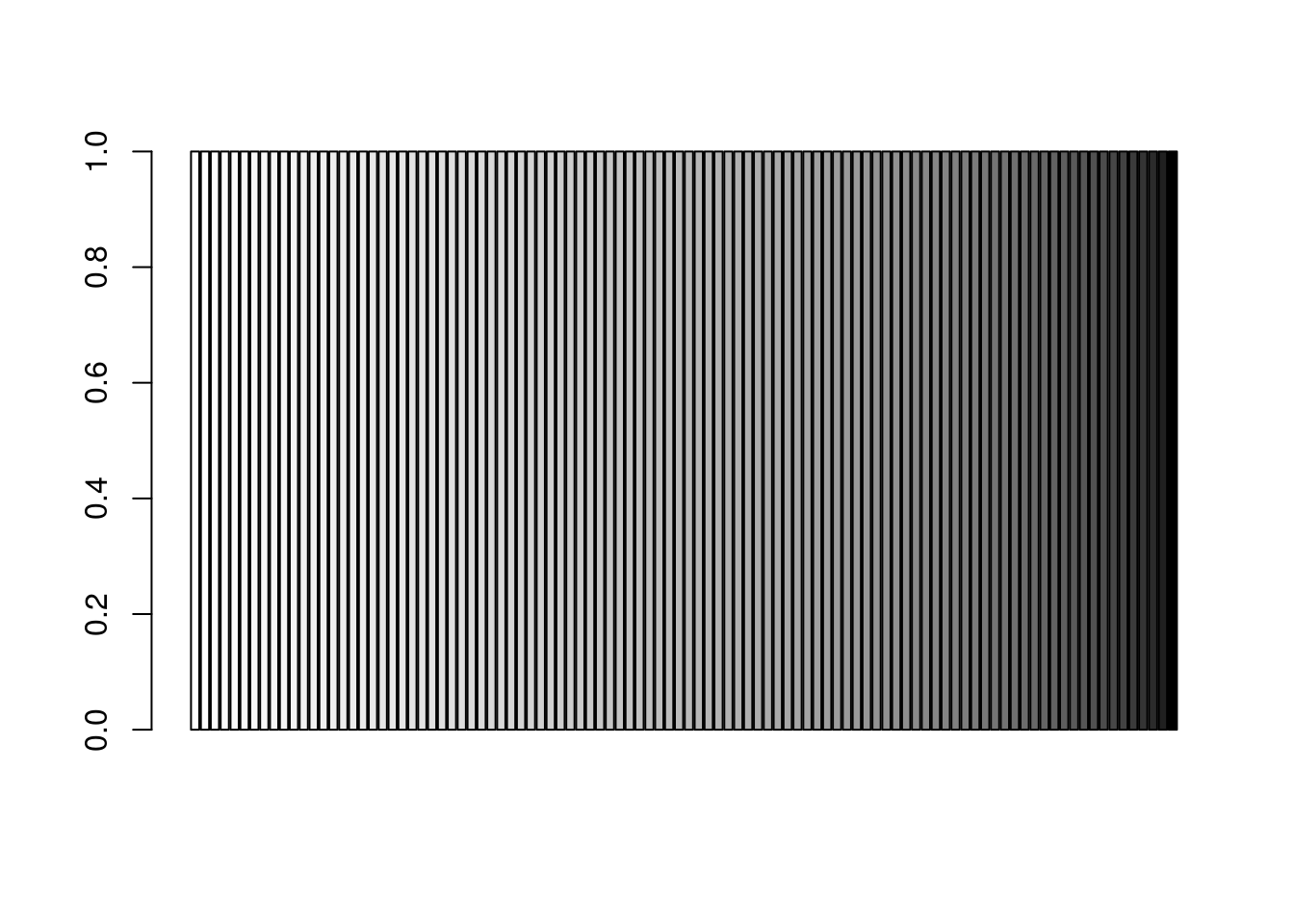``barplot(rep(1,50), col = gray.colors(50, start =1, end = 0))``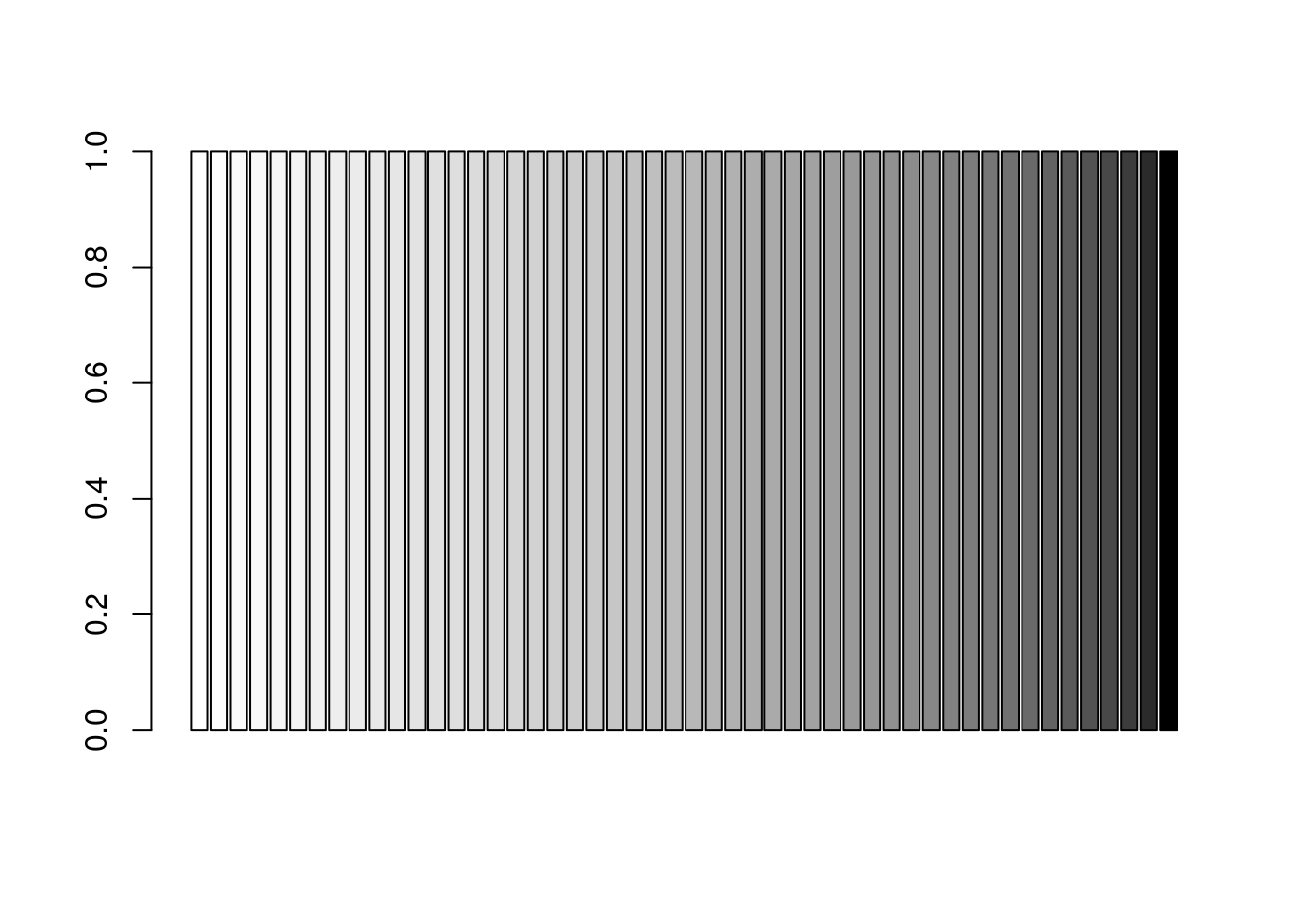``barplot(rep(1,25), col = gray.colors(25, start =1, end = 0))``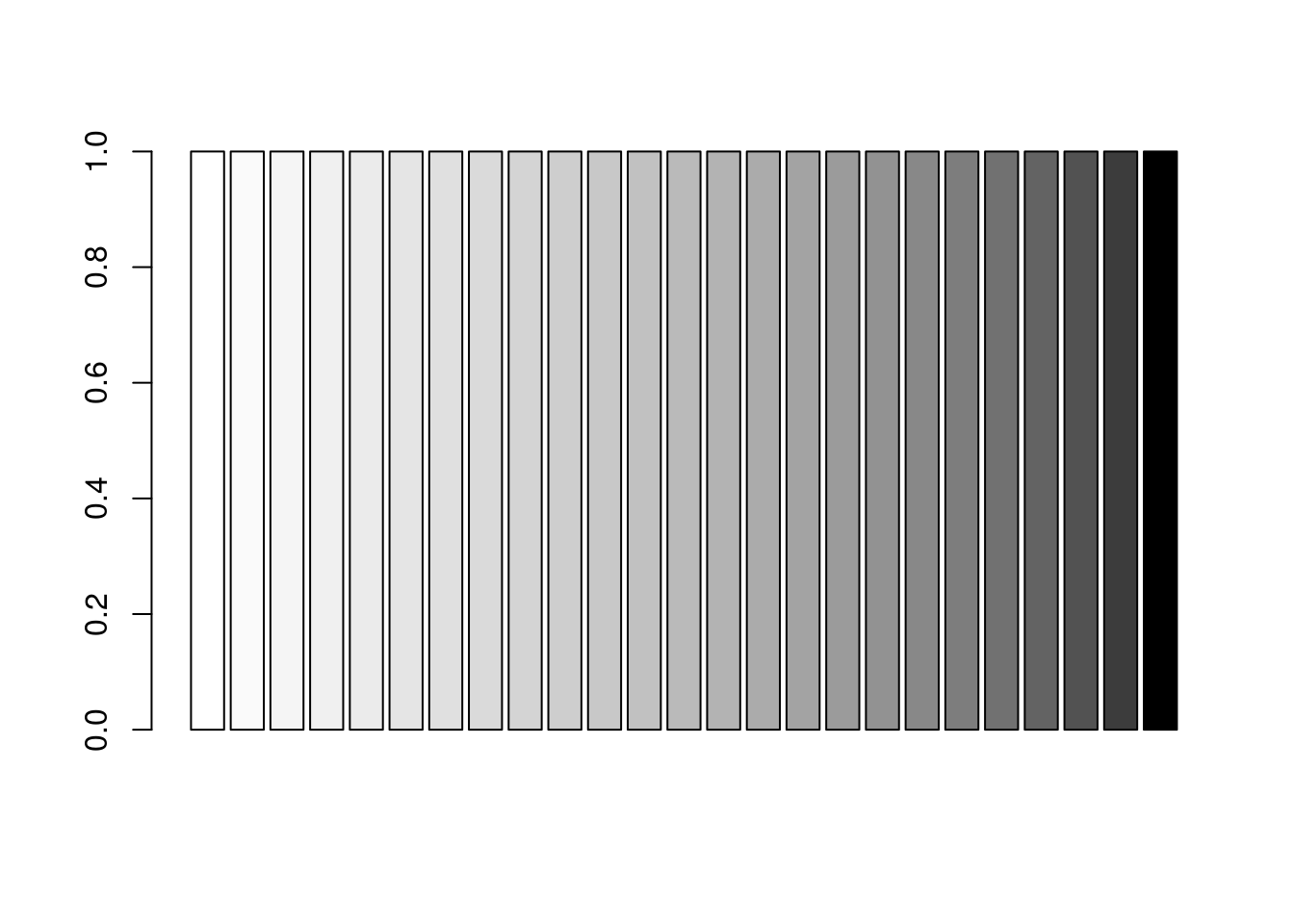``barplot(rep(1,10), col = gray.colors(10, start =1, end = 0))``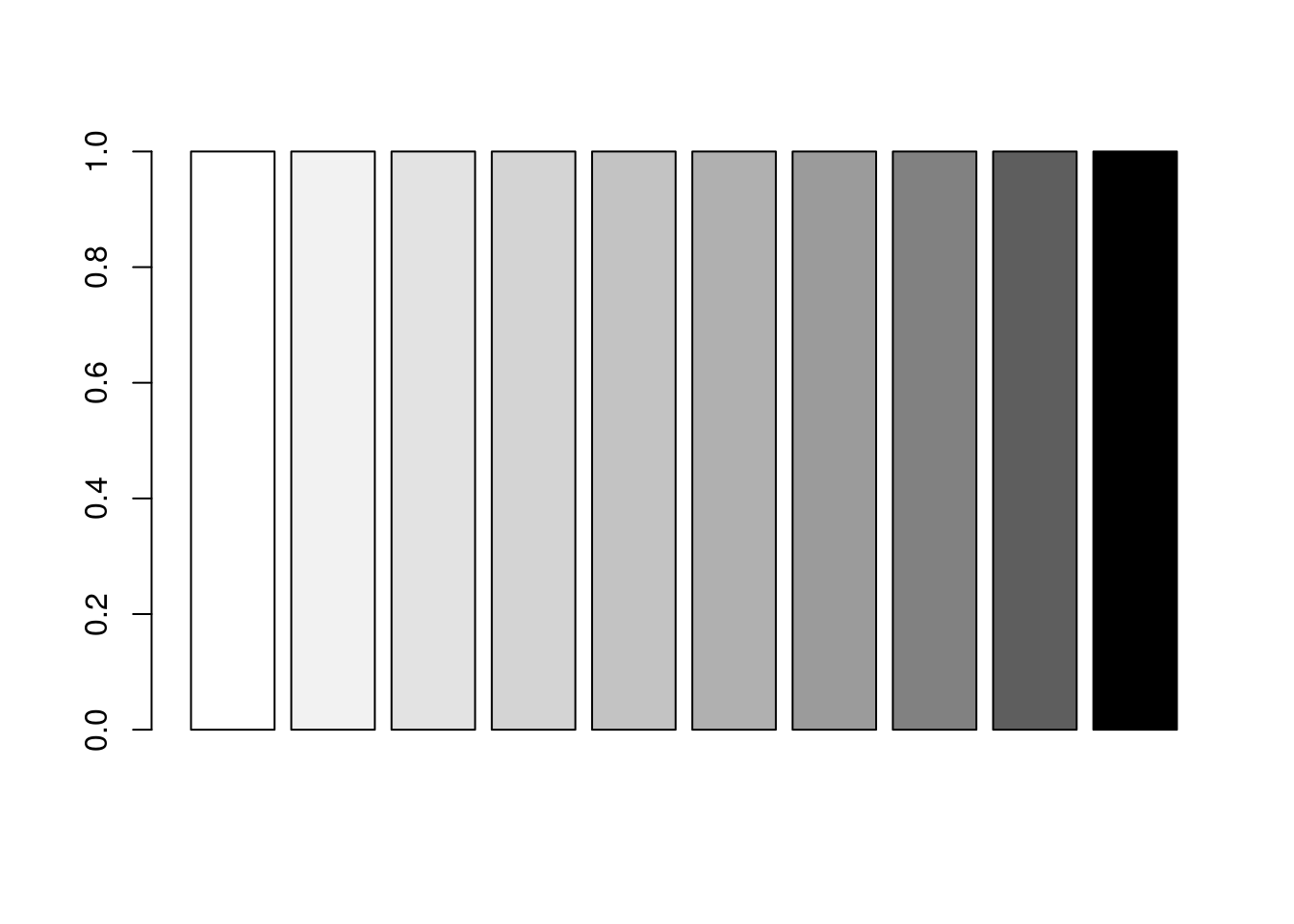# Creating a vector of heat colors

The `heat.colors()` function creates a vector of evenly-spaced red-to-yellow colors. The function has the form
`heat.colors(num_colors, alpha=value)`
alpha is an optional argument to specify alpha transparency of the colors.

``barplot(rep(1,100), col = heat.colors(100))``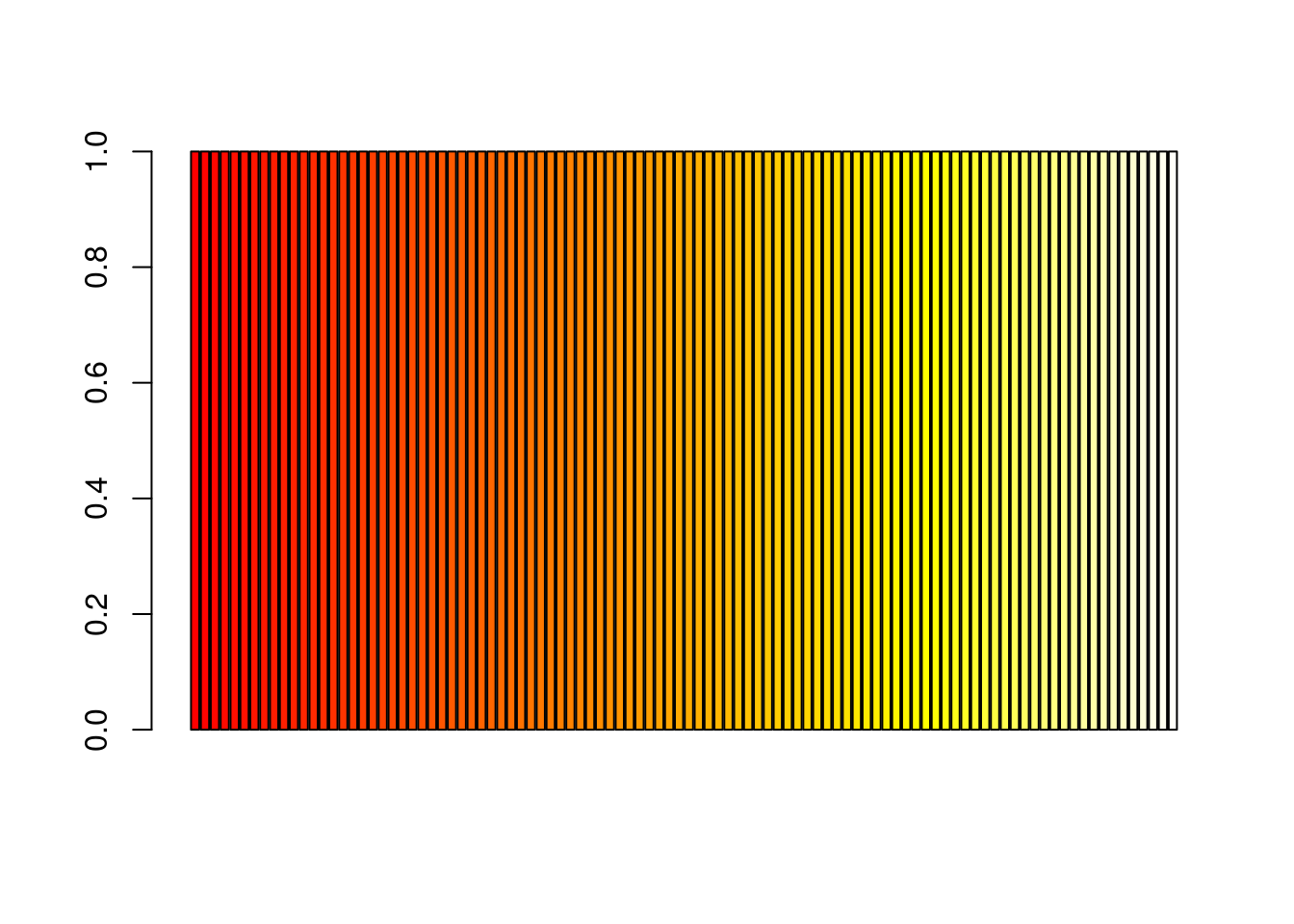``barplot(rep(1,50), col = heat.colors(50))``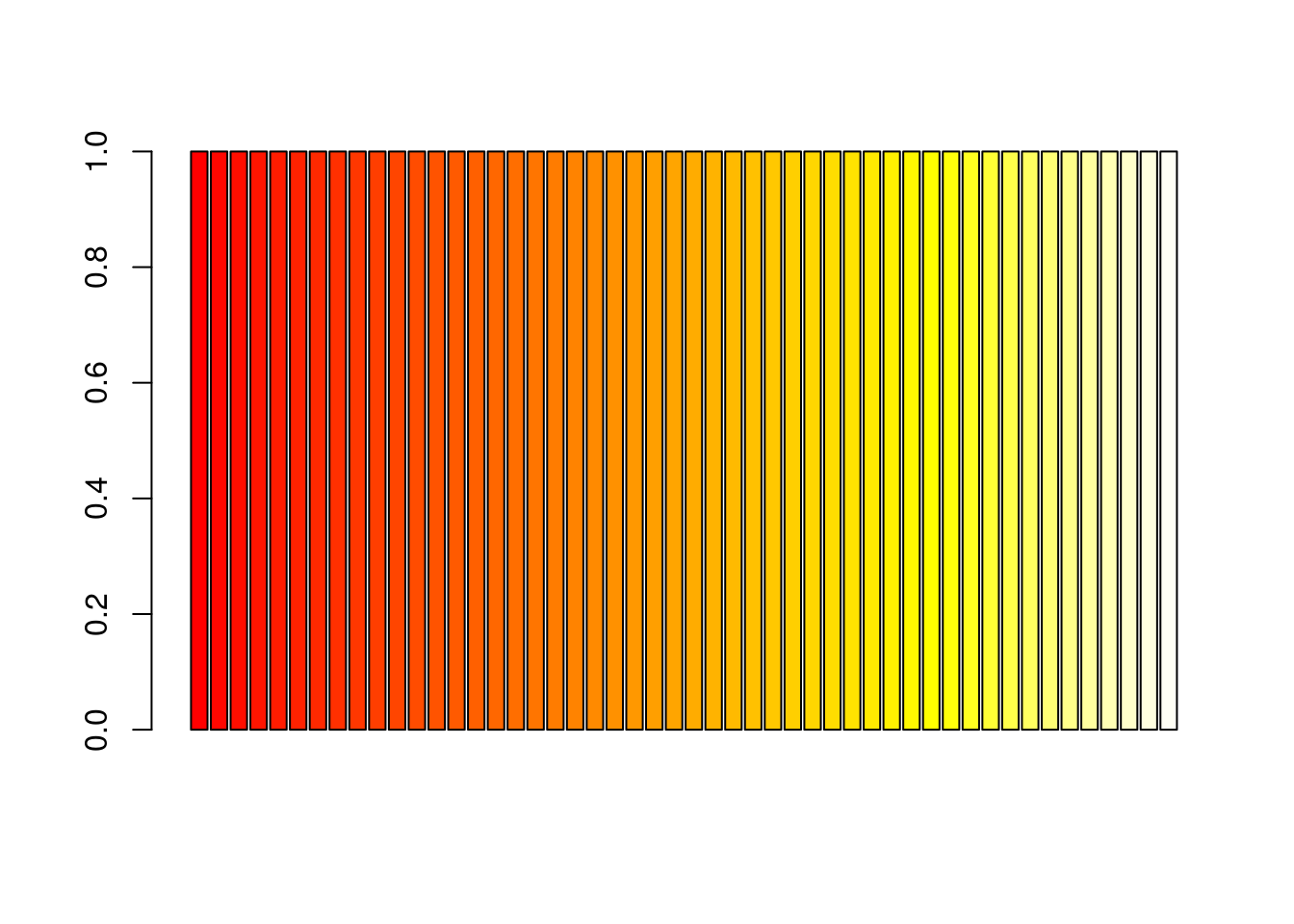``barplot(rep(1,25), col = heat.colors(25))``